The Australian Journal of Mathematical Analysis and Applications

 Home News Editors Volumes RGMIA Subscriptions Authors Contact

ISSN 1449-5910

Total of 16 results found in site

10: Paper Source PDF document

Paper's Title:

Solving Fractional Transport Equation via Walsh Function

Author(s):

L. M. F. N., Mathematics Department,
University of Setif,
Algeria
abdelouahak@yahoo.fr

Abstract:

In this paper we give a complete proof of A method for the solution of fractional transport equation in three-dimensional case by using Walsh function is presented. The main characteristic of this technique is that it reduces these problems to those of solving a system of algebraic equations, thus greatly simplifying the problem.

1: Paper Source PDF document

Paper's Title:

A New Family of Periodic Functions as Explicit Roots of a Class of Polynomial Equations

Author(s):

M. Artzrouni

Department of Mathematics, University of Pau
64013 Pau Cedex
Pau, France
marc.artzrouni@univ-pau.fr
URL: http://www.univ-pau.fr/~artzroun

Abstract:

For any positive integer n a new family of periodic functions in power series form and of period n is used to solve in closed form a class of polynomial equations of order n. The n roots are the values of the appropriate function from that family taken at 0, 1, ... , n-1.

1: Paper Source PDF document

Paper's Title:

Comparison Results for Solutions of Time Scale Matrix Riccati Equations and Inequalities

Author(s):

R. Hilscher

Department of Mathematical Analysis, Faculty of Science,
Masaryk University, Jan
áčkovo nám. 2a,
CZ-60200, Brno, Czech Republic.
hilscher@math.muni.cz
URL: http://www.math.muni.cz/~hilscher/

Abstract:

In this paper we derive comparison results for Hermitian solutions of time scale matrix Riccati equations and Riccati inequalities. Such solutions arise from special conjoined bases (X,U) of the corresponding time scale symplectic system via the Riccati quotient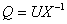. We also discuss properties of a unitary matrix solution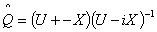of a certain associated Riccati equation.

1: Paper Source PDF document

Paper's Title:

Schwarz Method for Variational Inequalities Related to Ergodic Control Problems

Author(s):

Department of Mathematics, Badji Mokhtar University, Annaba 23000,
P.O.Box. 12, Annaba 23000, Algeria

signor_2000@yahoo.fr

Mcherihalima@yahoo.fr

Abstract:

In this paper, we study variational inequalities related to ergodic control problems studied by M. Boulbrachčne and H. Sissaoui , where the "discount factor" (i.e., the zero order term) is set to 0, we use an overlapping Schwarz method on nomatching grid which consists in decomposing the domain in two subdomains. For α ∈ ]0.1[ we provide the discretization on each subdomain converges in L -norm.

1: Paper Source PDF document

Paper's Title:

Weak solutions of non coercive stochastic Navier-Stokes equations in R2

Author(s):

Wilhelm Stannat and Satoshi Yokoyama

Technische Universität Berlin,
Strasse des 17. Juni 136, 10623 Berlin,
Germany.

The University of Tokyo,
Komaba, Tokyo 153-8914,
Japan.

E-mail: stannat@math.tu-berlin.de

E-mail: satoshi2@ms.u-tokyo.ac.jp

Abstract:

We prove existence of weak solutions of stochastic Navier-Stokes equations in R2 which do not satisfy the coercivity condition. The equations are formally derived from the critical point of some variational problem defined on the space of volume preserving diffeomorphisms in R2. Since the domain of our equation is unbounded, it is more difficult to get tightness of approximating sequences of solutions in comparison with the case of a bounded domain. Our approach is based on uniform a priori estimates on the enstrophy of weak solutions of the stochastic 2D-Navier-Stokes equations with periodic boundary conditions, where the periodicity is growing to infinity combined with a suitable spatial cutoff-technique.

1: Paper Source PDF document

Paper's Title:

Some Properties on a Class of p-valent Functions Involving Generalized Differential Operator

Author(s):

A. T. Yousef, Z. Salleh and T. Al-Hawary

Department of Mathematics,
Faculty of Ocean Engineering Technology and Informatics, Universiti Malaysia Terengganu,
21030 Kuala Nerus, Terengganu,
Malaysia.
E-mail: abduljabaryousef@gmail.com, zabidin@umt.edu.my

Department of Applied Science,
Ajloun College, Al-Balqa Applied University,
Ajloun 26816,
Jordan.
E-mail: tariq_amh@yahoo.com

Abstract:

This paper aiming to introduce a new differential operator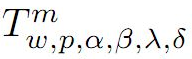in the open unit disc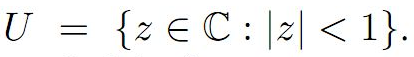We then, introduce a new subclass of analytic function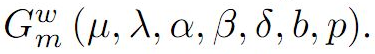Moreover, we discuss coefficient estimates, growth and distortion theorems, and inclusion properties for the functions belonging to the class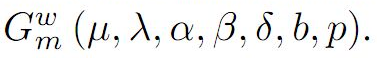1: Paper Source PDF document

Paper's Title:

A Note on Schur's Lemma in Banach Function Spaces

Author(s):

R. E. Castillo, H. Rafeiro and E. M. Rojas

Departamento de Matematicas, Bogota,
Colombia.
E-mail: recastillo@unal.edu.co

United Arab Emirates University,
Department of Mathematical Sciences, Al Ain,
United Arab Emirates.
E-mail: rafeiro@uaeu.ac.ae